All submissions of the EM system will be redirected to Online Manuscript Submission System. Authors are requested to submit articles directly to Online Manuscript Submission System of respective journal.

# Three-Dimensional Relativistic Shrinkage PK One-Dimensional Lorentz Shrinkage

Runsheng Tu*

Department of Chemistry, Huang gang Normal University, Hubei, China

*Corresponding Author:
Runsheng Tu
Department of Chemistry,
Huang gang Normal University,
Hubei,
China
E-mail: [email protected]

Received: 08-April-2022 Manuscript No. JPAP-22-60110-; Editor assigned: 13-April-2022 Pre QC No. JPAP-22-60110(PQ); Reviewed: 15-April-2022, QC No. JPAP-22-60110; Revised: 18-April-2022, Manuscript No. JPAP-22-60110(A) Published: 22-April-2022, DOI:10.4172/2320-2459.10.3.005

Visit for more related articles at Research & Reviews: Journal of Pure and Applied Physics

## Abstract

Ignoring the internal structure of moving objects and treating them as rigid bodies is not only out of practice but also inconsistent with the spirit of scientific exploration. To change this status quo, consider the relativistic effects of real object motion. Consider the mass-velocity relationship as an initial mechanism to discuss the effect of velocity on the space around an object and on the volume of the object. A series of new conclusions are obtained, such as "the space distortion of a moving system with mass due to inertial motion at ultra-high speed, and even the generation of neutron like stars or black holes", and the 3D contraction of moving objects due to inertial motion, which can oppose the corresponding old views. This confrontation threatens the status of the theoretical criterion "covariant under Lorentz transformation", and thus has a great impact on the whole field of theoretical physics.

#### Keywords

Relativity; Rigid bodies; 1D Lorentz contraction; Mass-velocity relation; Three-dimensional relativistic contraction

#### Introduction

Special relativity uses the Lorentz transformation to discuss changes in space due to motion. To ignore the physical mechanism of this change is to ignore the internal composition and structure of the moving object and treat the moving object as a rigid body. After Einstein, no one supplemented the exploration of the physical mechanisms by which objects contract due to motion. This paper also refers to the space contraction derived from the Lorentz transformation as the Lorentz contraction (it's a one-dimensional contraction of space). Since the Lorentz contraction is a contraction of mathematical reasons and does not require a physical mechanism for the contraction (a contraction that takes into account a specific contraction mechanism is not a Lorentz contraction), therefore, people who do not want to deny the Lorentz contraction never discuss "not to What are the consequences of treating a moving body as an approximate rigid body".

The value of theories is that they function in practice, and in practice objects in motion have internal structures. It can be seen that under the premise that the internal composition and structure of moving objects cannot be ignored, the practice of special relativity intentionally deviates from reality (ignoring the internal composition and structure of objects that should not be ignored), which violates the principle of reality and is also inconsistent with the spirit of scientific exploration.

#### Materials and Methods

There are various factors that can cause the volume contraction of moving objects to deviate from the Lorentz contraction. Unfortunately, people also seem to forget to discuss the role of various contraction factors. The consequences of the clock's high-speed movement (even faster than the speed of light) have been discussed by many . However, in the case of ultra-high speed inertial motion, how the volume of the object and the space around the object change is hardly discussed. Around the world, many people have exposed the difficulties of relativity [2-15]. However, before this article, the critics mainly exposed the logical contradictions in the special theory of relativity (as if caught in this quagmire and unable to extricate themselves). We have also demonstrated that space shrinking due to motion is not relative [16-18] and the necessity of establishing theory of relative-absoluteness [18,19]. Now, I seem to have jumped out of this quagmire and discovered a new pattern by filling in the gaps. Looking back at the shortcomings of special relativity, the effect is particularly good. Reference  mentions one factor that affects the stereoscopic contraction of moving objects, and only involves linear contraction and does not discuss the nonlinear changes in space-time (and the case of ultra-high speed is not discussed). Here we want to expand to multiple influencing factors and discuss non-linear changes in space-time. In this paper, the mechanism and results of the three-dimensional contraction and four-dimensional spatiotemporal changes of the mass system due to high-speed motion will be introduced in detail. For criticizing the theory of relativity and quantum mechanics, many scholars have suffered from aesthetic fatigue, so they ignored the remarks criticizing the pillar theories . Hopefully the reviewers will be shocked and relieved of fatigue when they see this article.

The physical mechanism by which the volume of a non-rigid body shrinks in all directions due to motion

The relativistic mass-velocity relationship is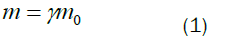Here,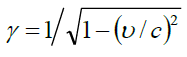there are various derivation methods for Equation (1), which are not exclusively derived from the theory of relativity [18-21]. Special relativity ignores the internal composition and structure of moving objects and treats them as rigid bodies that cannot deform, and admit that objects traveling very close to the speed of light will shrink into very thin panels. The reason for this change is also believed to be the "contraction of space due to motion" causing the objects embedded in it to contract. However, from Equation (1) and general relativity, it is easy to see that when an object moves very close to the speed of light (we will calculate this speed limit in Section 2.3, and the speed greater than this value but less than the speed of light is called super high speed), it can become a spherical black hole, and the space around the object is also will bend badly. This means that the space around the mass carrier is bent by the movement of the mass carrier. When the space is curved, the volume of the moving object shrinks, not only in the direction of motion, but also in other directions, and the multiple of shrinkage is not always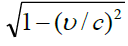times (the volume of a moving object shrinks, but the space just bends instead of shrinking). This conclusion, which takes into account the general theory of relativity, is in serious conflict with the special theory of relativity that "space contracts in a single stretching direction due to motion". There is no reason to suggest that for the contraction of a moving physical ruler (or the change in space caused by a moving object), general relativity effects and special relativity effects work separately, and then can be superimposed linearly (on the contrary, to think so without a reason is to subjectively separate general and special relativity). Previously, the way to avoid this contradiction was to ignore the internal composition and structure of moving objects, or to ignore general relativity effects of particles inside moving objects (i.e. ignoring gravitational interactions inside moving objects). However, for the discussion of changes in moving objects, there is an overlap between the scope of application of general relativity and that of special relativity. In addition, the set condition is that the mass carrier has high-speed motion within the framework of special relativity. In this overlapping area of application, the relativistic effect of 3D velocity still exists even if the concept of 4D velocities is used.

As mentioned above, for the volume of the object describing the motion to shrink due to the motion, the conclusion of the special theory of relativity contradicts the effect of the general theory of relativity obtained using Equation (1). This contradiction cannot be completely resolved by dividing the speed range and taking an approximation. The Lorentz transformation is widely used by special relativity. Its popular form is as follows: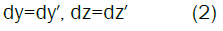According to it, the expression for the contraction of the ruler moving in the X-axis direction due to the movement is as follows: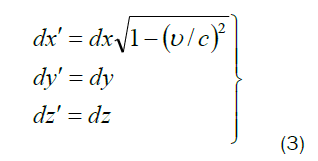The moving ruler does not contract in the Y-axis and Z-axis directions perpendicular to the velocity. For convenience, we call the contraction expressed by Equation (3) "derived from the Lorentz transformation" as the Lorentz contraction (or one-dimensional relativistic contraction or Lorentz length-contraction). If a box is moving, Lorentz contraction means that one side of the box is shortened. Let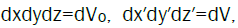the Lorentz volume shrinkage formula obtained from Equation (3) is: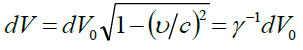Under certain circumstances, the definite integral of this formula can be obtained (the integral range is from the origin to the finite value), turn out: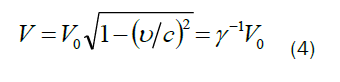Equation (3) or Equation (4) is obviously a 1D contraction-formula of space. They are completely a mathematical result, and the expressed contraction has no specific physical mechanism (movement is only the theoretical cause of contraction rather than a specific physical mechanism). Einstein seems to have no subjective desire to discuss this physical mechanism (the characteristics of the theory also determine that he cannot discuss it). On the premise of not exploring the physical mechanism of space contraction, the special theory of relativity simply treats moving objects as non-deformable rigid bodies without internal composition. Only in this way can the contraction of the moving object in the direction of movement be attributed to the contraction of space due to the movement (that is, the difference between the three-dimensional contraction of object volume and the one-dimensional contraction of space is erased). However, the relativistic effect of particles inside an object has a clear physical mechanism. Can it be ignored? After reading the discussion about the influencing factors of the volume of moving objects below, we can judge correctly.

Conservation of orbital angular momentum

The arguments here leave traces of the old quantum theory. The Bohr model of the hydrogen atom in the old quantum theory is partially compatible with modern quantum mechanics. In addition, using Bohr's planetary model makes it easier to understand the physical mechanism of how objects contract due to motion. The key is that the old quantum theory is a better excess theory. As a transition, Bohr model can be used.

When the ground state hydrogen atom moves, the mass m of 1s electron increases according to the law of Equation (1). The orbital angular momentum of Bohr hydrogen atom is expressed as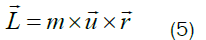For the ground state hydrogen atom, according to the planetary model, we have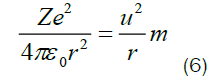Here, Z is the effective nuclear charge, and u is the electron orbital velocity. Substituting the scalar form of Equation (5) with mur=Ñ into Equation (6), we can obtain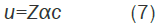Here, α is the fine structure constant, and its value is about 1/137. Equation (7) and its derivation process show that when the mass of the electron is changed by the overall motion of the hydrogen atom, the speed of the planetary motion of the electron remains unchanged. As long as the orbital angular momentum of the 1s electron is conserved, when the electron mass m increases, the orbit radius r becomes smaller. Comparing three Equations (1) and (7) and mur=Ñ, we have Equation (8).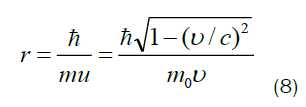Here, υ-the velocity of the hydrogen atom, L-the orbital angular momentum of the hydrogen atom and m0-the mass of the electrons in the static hydrogen atom, m-the electron mass with an overall motion on the basis of the electron dynamic mass in the hydrogen atom. Different states of electron mass can be distinguished by adding subscripts: me is the mass of the stationary electron, m0 is the mass of the electron in the stationary hydrogen atom, and m is the mass of the electron in the moving hydrogen atom. If you seek more accuracy, you can use the reduced mass of electrons.

Using the solution of the Schrödinger equation, the more reliable conclusion that "the radius of the moving hydrogen atom decreases" can be obtained. In quantum mechanics, the size of an atom is a constant of its radius. As the atomic radius shrinks, the atomic volume shrinks in three dimensions.

The mass-velocity relationship and the solution of the Schrodinger equation together determine that the radius of the hydrogen atom decreases as the electron mass increases

Solving the Schrodinger equation of hydrogen atom can get the Bohr radius expression of hydrogen atom.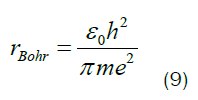Comparing Eqs (1) and (9), we can obtain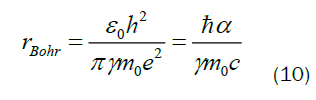can be seen from Equation (10) that when the hydrogen atom moves, the electron mass increases, the Bohr radius decreases, and the hydrogen atom shrinks in all directions. For the covalent molecule H2, the bond length of the chemical bond is also proportional to the Bohr radius or the size of the hydrogen atom. In this way, a hydrogen ruler composed of hydrogen molecules will also shrink in all directions due to motion. The expression for the relativistic contraction of the volumes of hydrogen atoms and molecules is as follows: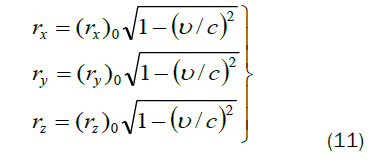Here, ri is the radii in the three mutually perpendicular directions of the moving hydrogen atom, and (ri) 0 is the radii in the three mutually perpendicular directions of the stationary hydrogen atom. The volume of hydrogen atom V= (4/3) πr3. Therefore, we have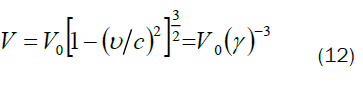Here,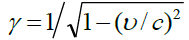V0 is the volume of stationary hydrogen atoms or hydrogen molecules, and V is the volume of moving hydrogen atoms or hydrogen molecules. Note: The exponent on γ in the Lorentz contraction formula of volume is -1 (see Equation (4) for details). Equation (12) shows that the shrinkage of the volume of an object due to motion is not limited by the direction of motion. In the process of deriving Equation (12), the massvelocity relation of special relativity and quantum mechanics effect are used but the gravitational effect is ignored. Under the condition that the speed is not particularly high, the general relativity effect is too weak to be ignored compared with the quantum mechanical effect. The Schrodinger equation utilized is a linear equation. Therefore, the shrinkage discussed in Sections 2.1 and 2.2 is still a linear shrinkage of volume. Equations (11) and (12) express the three-dimensional shrinkage of the atomic volume. They are quantitative relationships between volume and velocity that are applicable within a certain range. Previously there was only a one-dimensional Lorentz contraction expression, generally called the length-velocity relationship. Previously only knowledge of Lorentz onedimensional contractions (commonly called the length-velocity relationship) was known.

For the covalent molecule H2, the bond length of the chemical bond is also proportional to the Bohr radius or the size of the hydrogen atom. In this way, the hydrogen ruler composed of one hydrogen molecule will also shrink according to the law of Equation (12) due to the movement (the speed of the Hydrogen ruler limited to close to the speed of light and not very close to the speed of light).

General relativity effects due to inertial motion

It has been experimentally confirmed that the inertial mass of moving particles increases due to motion. Considering that the inertial mass is equal to the gravitational mass, we can be sure that the gravitational force of the moving particle will also increase due to the motion (space around particles can be distorted by motion). As the speed of the object increases, the mass of the particles inside it increases. The first effect that should not be ignored is that the gravitational force between particles in the object increases and the distance decreases (if the speed is lower than this, the inter-particle attraction in the atom can be ignored). When the velocity increases again, the distortion of space-time caused by mass becomes more obvious, and even the atom and the object can collapse.

We discuss the quantitative bounds of these two effects using the example of a moving hydrogen atom. The velocity of the hydrogen atom required for the gravitational force between the nucleus and the electrons outside the nucleus to reach 1/100 the electromagnetic force can be calculated. The electrons in it have two levels of motion (except spin motion): Orbital motion of electrons (speed recorded as u); electrons move with the motion of hydrogen atoms (speed recorded as υ). Since u=Zαc, the difference between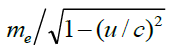and me is only 0.3/10000, and the relativistic effect of electron motion at this level can be ignored. According to this condition, we have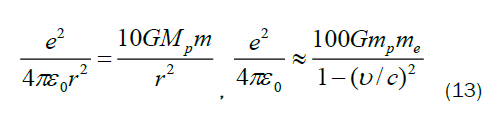Here, G is the gravitational constant; mp is the stationary mass of the proton,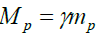is the mass of the proton in the moving hydrogen atom, m is the mass of the electron in the moving hydrogen atom, me is the mass of the stationary electron, and υ is the movement speed of the hydrogen atom. Substitute the corresponding constant into Equation (13), we can obtain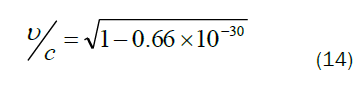This value is 0.999... 9 (there are 30 consecutive 9). Speeds that reach or exceed this value can be called hyper speed. When the speed of the hydrogen atom reaches this value, the gravitational force between the nucleus and the electron can obviously affect the size of the hydrogen atom, which should not be ignored (the change of the space-time curvature outside the nucleus should not be ignored). "The size of the hydrogen atom shrinks due to motion" caused by this relativistic effect will obviously deviate from Equation (12). The velocity expressed by Equation (14) is the lower limit of the velocity of the gravitational force between particles inside the object that should not be ignored, and also the lower limit of the velocity that the space-time distortion effect should not be ignored.

Schwarzschild radius is rg=2 Gm/c2. It shows that the mass increases, the event horizon of the black hole increases, and the possibility of a finite mass object becoming a black hole increases. As the mass of each atom continues to increase, the moving object will be compressed to a small volume by gravity. As the horizon determined by the mass of matter within this small volume continues to grow, so that an occupied space containing the entire object can be reached. At this point, the object becomes a standard black hole (Objects collapsed and deformed before reaching the density of a neutron star, and living things died long ago).

The same is true for the conclusion of the quantitative analysis below. By substituting the relativistic mass velocity relationship into the Schwarzschild radius expression, we can obtain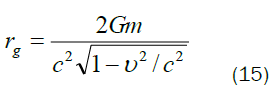The speed required for a hydrogen atom to become a black hole with an event horizon radius of the same order of magnitude as the Compton wavelength of a neutron or proton is υ.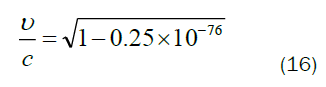This ratio is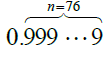i.e., the υ is very close to the speed of light. It is the lower limit of the velocity at which hydrogen atoms collapse due to motion. The situation is similar for other objects moving at high speed. Special relativity just doesn't allow objects to travel up to the speed of light. Therefore, the above very close to the speed of light is still within the allowable range of special relativity. It can be seen that moving rulers or rods or objects cannot be regarded as rigid bodies and ignore the relativistic effects of particles inside them. When the speed of motion of an object is very high, the contraction of its volume due to motion cannot be explained by the contraction of space due to motion.

The mass of one kilogram of matter increases due to motion to reach an event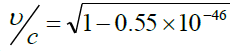this ratio is about 0.999•••9 (there are 46 consecutive “9”). This velocity value is the lower limit on the velocity at which the object collapses due to motion.

In this section, within the framework of the special theory of relativity, as the speed of the object increases, the gravitational interaction (the effect of general relativity) inside the object cannot be ignored. The gravitational effect mentioned here mainly refers to the collapse of the object caused by the ultra-high-speed motion of the object into a neutron star or a black hole, and the curvature of the space around the object. Gravitational contractions caused by objects moving at low speeds can generally be ignored. Equation (12) is the standard expression of the relativistic effect of a moving non-rigid body. Equation (14) shows that when the speed of hydrogen atom is less than that indicated by Equation (14) (the gravitational force between particles inside the object is much smaller than the electromagnetic force), Equation (12) is applicable to atoms, molecules and dense metal substances. "The volume of other substances shrinks due to movement" will deviate from Equation (12). Although the discussion in this section does not give an expression for the shrinkage of an object due to the non-negligible gravitational force between particles inside, it has been clearly pointed out that such shrinkage exists through quantitative and qualitative analysis. It belongs to the general relativity effect induced by the inertial motion. The reason is that the relativistic effect that has the participation of gravity or the consequence of space-time distortion is the general relativistic effect, and it is a nonlinear relativistic effect. Although the initial inducements of the linear relativistic effects and nonlinear relativistic effects discussed in Section 2 are inertial motions, these two contractions are independent of each other, and they have no logical relationship with the Lorentz contraction. From a qualitative point of view, the closer the speed of an object is to the speed of light, the greater the curvature of the space around the particles inside the object, and the object can even shrink to the extreme-turning into a neutron star structure or collapsing into a black hole.

Although both the special theory of relativity and the general theory of relativity use the changes of space-time to describe the effect of force, for describing specific high-speed moving objects with internal composition and structure, the effect produced by the physical mechanism of general relativity and the effect produced by the mechanism of special relativity are contradictory of: The mathematical conclusion that there is no specific mechanism in the special theory of relativity is that the moving ruler (or space) shrinks only in the direction of motion (1D contraction of space or volume. The space before and after shrinking is linear), and the object will not collapse due to motion; the effect of the mechanism of general relativity is that the inner and outer space of the moving ruler is curved (The contraction of the corresponding object due to motion is also inconsistent with the conclusion of special relativity-it is a three-dimensional contraction of space, and can collapse due to hypervelocity motion. At the same time, the linear space becomes a nonlinear space due to high-speed motion). The contraction mechanism revealed in this section shows that, for the consequences of the mass carrier motion, the speed ranges of "the special relativity 'mechanism' taking effect and the general relativity mechanism taking effect" are completely coincident. Taking approximations can stretch its applicability a bit, but not completely. For example, for hydrogen atoms and solid hydrogen to contract due to motion, the velocity interval where the special relativity effect and the general relativity effect are applicable together is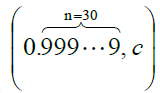(The reason is that, as far as the speed condition is concerned, as long as the speed is not greater than or equal to the speed of light, the theory of relativity applies). Can two types of relativistic effects acting on the same object be superimposed linearly? The gravitational contraction effect, the Lorentz contraction effect and the pure mass contraction effect cannot be superimposed linearly. In this common applicable range, there is a speed interval in which neither the general relativity effect nor the special relativity effect can be ignored. Another contradiction between special relativity and general relativity is that for an accelerating system, within the framework of general relativity there is one system, while within the framework of special relativity there are multiple systems. No matter how much approximation is taken, this contradiction cannot be eliminated.

Influence of van der waals forces

In the case of a ruler made of solid hydrogen, it contracts due to motion, involving changes in the distances between molecules. The van der Waals force between molecules is still an electromagnetic force in nature, and the bonding electrons are also bound electrons. The mass of the bound valence electrons changes while the charge of the electrons and nuclei remains the same, and the distance between the molecules will be shortened.

Reduced vibrational frequency of ions in ionic compounds

For ionic compounds, the mass of the ions at each lattice point within the crystal increases due to motion. In this way, the vibration frequency of the ion is reduced (the reason is that the vibration of the ion is a reciprocating motion, and the state of motion needs to be changed continuously, and the increase in mass makes it more difficult to change the state of motion), and the volume of the ion and the volume of the crystal will decrease accordingly. The volume of the crystal shrinks in all directions. Molecular thermal activity in liquid and gaseous substances also decreases as the molecular mass increases.

The entropy of an adiabatic system is reduced by motion

For a closed system, if its volume decreases, its entropy will inevitably increase. On the contrary, its entropy decreases and its volume must decrease. For adiabatic non-solid matter, the mass of its components increases, the mass of particles increases, the thermal motion activity decreases, the degree of disorder of the system decreases, the entropy decreases, and the volume decreases. In short, when the mass of the molecules in the gas or liquid increases due to motion, the thermal motion of the molecules decreases, their entropy decreases, the distance between molecules decreases, and the volume decreases.

Sections 2.5 and 2.6 describe the thermodynamic mechanism of the contraction of matter due to motion. The shrinkage it causes is also three-dimensional volume shrinkage.

To sum up, for objects composed of a large number of molecules or ions to contract due to motion, even though quantitatively there is a difference from Equation (12), qualitatively they all contract in all directions due to motion. The thermodynamic mechanism in the above contraction mechanism is obviously also a physical mechanism for the lifespan extension of the moving organisms. That is, the physical mechanism by which a moving mechanical clock slows down. These factors (physical mechanisms) act simultaneously on a moving object. It's just that for objects of different natures, different factors play different roles

The difficulty of accelerating infinite space and the difficulty of the contraction center of infinite space contracted due to motion

All four dimensions in the continuum of four-dimensional space can be extended infinitely. Relativity holds that every moving object is associated with its own frame of reference. The space of these frames of reference is infinite. There seems to be no problem with this concept. However, if we study it carefully, we will have the problem of being divorced from reality. For example, when we accelerate a stationary fine needle B in the A system, according to the theory of relativity, B must be extracted from the space in the A system with an infinite space and accelerate with B. In this case, the process of accelerating a stationary object is also the process of infinite space reproduction. Who can guarantee that such spatial reproduction is real? Is there any reason to say that infinite space can reproduce? Recognize that an object is associated with a system of motion, and each system of motion has an infinite space. There are many objects in different motion states in the real space, and there are many different infinite spaces. Since there is only one real cosmic space, those infinite spaces associated with moving objects can only be virtual to satisfy theoretical needs.

On the premise that each moving object has its own infinite space, accelerating the object is to accelerate the infinite space. There are also logical problems in acknowledging that a real cosmic space contains many infinite spaces. There are difficulties in accelerating an infinite system and its infinite space. Axioms and special relativity tell us that an infinite object cannot be accelerated. Otherwise, we must admit that there is instantaneous action at a distance, or directly admit that the infinite space separated from the static space is a virtual space (denying that space is a real object), or denying the materiality of space. A system equivalent to a gravitational field is a continuously accelerated system. The continuous acceleration of space also has the problem of shrinking centers. Space shrinks continuously due to continuous acceleration. Where is the center of its shrinkage? If the object B in the above example is not at the center of the universe, what reason do we have to say that the shrinking center of space is exactly at the center of B? If the shrinking center of the infinite space is not at the center of B, then the infinite space shrinks a little bit, and B embedded in the space will not know where to go. But the reality is not like that. As a complete theory, the theory of relativity should make clear regulations or explanations to the problems mentioned above. In short, if the movement of space is not real, the contraction of space due to movement is also not real.

#### Results and Discussion

If the Lorentz contraction coexists with the contraction caused by the contraction factors described in Section 2, then the experimentally measured contraction of the moving rod should be a double contraction in the direction of motion. However, no experiment detected a double contraction of the moving rod. Therefore, only one of these two types of contractions is true (we can only choose to believe one of these two types of contractions). The following discussion will help us make the right choice. The following factors determine our preference for 3D shrinkage effects (including spatiotemporal changes determined by general relativity mechanisms). That is, choosing to believe that "three-dimensional shrinkage" (including the four-dimensional space-time distortion also caused by inertial motion) in the big PK described in the title of this article is the winner.

(1) There are more subjective factors or hypothetical components of contraction without a clear physical mechanism.

As long as the relativistic mass velocity relationship and the theory of neutron stars and black holes are correct, it is true that when observing an object or system moving at a super high speed in a stationary system, the object can collapse due to motion or the space in the system is bent due to motion. Once a moving object collapses (space-time has undergone non-linear changes), it must not return to the state before the collapse after it stops moving. Especially after the organism has undergone the changes of body collapse, it cannot return to the previous state of biological activity. Objects within the framework of special relativity change due to motion, no undeniable determinants can be found (All that can be found is Einstein's subjective understanding of the Lorentz transformation).

(2) General relativity can replace special relativity, but the reverse is not true.

Special relativity is an approximation of general relativity. However, the reverse is not true.

(3) Formally, the three-dimensional contraction derived from the mechanism of general relativity includes the one-dimensional contraction derived from the mathematical reasons of special relativity

For example, the content of Equation (11) includes the content of Equation (3). But the reverse is not true.

The following are supplementary instructions.

The equivalence of different inertial frames and the Lorentz contraction characteristics under Lorentz transformation also require that when the inertial frame is in inertial motion and observing in the system, all kinds of things in the system will not change substantially, especially the creatures in the system cannot feel abnormal. The special theory of relativity does not allow the phenomenon of "objects collapse due to inertial motion". General relativity allows such nonlinear changes in space, which is a negation of the view of special relativity. If the mutual observation is that the observed mass system collapses due to motion, it will definitely cause the material system of the entire universe to truly collapse after an object reaches very close to the speed of light, and this consequence is impossible. Therefore, the conclusion that "the mutual observation is the contraction of the ruler and the increase of the mass in the observed system" cannot be established. The Lorentz transformation cannot be used as a mathematical tool to describe the real space-time without ignoring the effect that space-time is distorted by motion and even objects are collapsed by motion. It is revealed here that it is the "logical cycle of mutual collapse of super-high-speed relative moving objects" caused by the principle of relativity in the special sense. Hubble's law states that the farther away the galaxy is, the higher its velocity relative to us. The same is true when looking at the center of the universe (or the center of total galaxies). The fact that the expansion of the universe is accelerating can also weight this conclusion. The fact that the expansion of the universe is accelerating can also weight this conclusion.

Lorentz contraction (one-dimensional contraction) has no specific physical mechanism. It is caused by mathematics. The contraction discussed in Section 2 of this paper is contraction with clear physical mechanisms, and these mechanisms are obtained according to the basic principles of relativity (to deny the contraction mechanism and its application effect derived in this paper is to deny the theory of relativity). The second section reveals that the Lorentz contraction in the context of special relativity is a virtual contraction of space. Section 3 reveals that the motion of space is virtual motion of virtual space.

The moving ruler or rod used for the experiment is a real entity with internal composition and structure (usually alloys). Therefore, the experimentally measured contractions that "must be explained by the contraction of the length of the rod due to motion" should all be the contractions described by Equation (11) or (12). The Lorentz contraction described by Equation (3) can at most be the result of the induction and abstraction of the contraction caused by the contraction mechanism introduced in Section 2. However, contractions "caused by the contraction mechanism described in Section 2" are omnidirectional contractions, whereas Lorentz contractions are contractions in a single direction of extension. It can be seen that the Lorentz contraction described by Equation (3) is not the result of induction and abstraction of the contraction caused by the contraction mechanism in Section 2. The Michelson-Morley experimental phenomenon should be explained by the constant speed of light.

There is only one real universe. However, the special theory of relativity believes that each inertial motion object corresponds to a motion system, and the space of each motion system is an infinite space. If there is no difference between the real space and the space determined by the special theory of relativity, then it is very difficult for many infinite spaces to interlace with each other (not only the number of contradictions). It can be seen that there should be a difference between the real cosmic space and the theoretical space associated with objects moving in a straight line at a uniform speed. Since there is only one real cosmic space, other spaces can only be theoretical spaces (i.e., virtual spaces). The space that can be accelerated and can be contracted by motion is also the theoretical virtual space (this conclusion can solve the contradiction disclosed in Section 3). As mentioned above, "theoretically, the selected motion system can move together with its own space, and such space shrinks due to motion (not the contraction of physical mechanism)" is not a conclusion that can stand various tests. The connection between the four-dimensional space-time continuum and real space-time is in doubt.

"Mutual observation is the contraction of the ruler in the observed system", there will be logical contradictions. There is also a logical contradiction in "mutual observation is the slowing of the clock in the observed system". This is determined by the lack of authenticity of space-time in Lorentz transformation (and explanation of "relativity principle of Lorentz transformation"). The two-way round the world navigation experiment of atomic clock confirms that the moving clock slows down, but it cannot be used as the experimental evidence that "if the two systems of relative motion observe each other, the conclusion is that the observed clock slows down ". On the contrary, the experimental results are only beneficial to the conclusion that the slowing down of the moving clock is unidirectional. Because the pilot on the plane cannot observe that the clock on the ground is slower than the clock on the plane. We can also find cases that corroborate with the relativity problem of relativistic space contraction. Suppose pair of twin brothers is born at the same time when inertial frames D and E meet, and fall on D and E respectively. The brothers have been playing video calls while D and E are moving away from each other. Under the premise of deducting the propagation time of electromagnetic waves, the brothers found out who ages faster through video? Special relativity cannot answer this question. This shows that there is an insurmountable contradiction in the relativity of the clock being slowed down by motion, and the mathematical results of the time transformation of the Lorentz transformation will appear logically contradictory (i.e., inconsistent with reality) in some cases.

As long as the relativity of time delaying due to motion is contradictory, there must be a problem with the relativity of space shrinking due to motion.

The time and space in the system are not relative due to the movement of the system. Lorentz contraction in a single direction of extension does not match the fact that the volume is contracted in all directions by motion. This shows that the Lorentz transform is just a mathematical tool. The status of the theoretical criterion "covariant under Lorentz transformation" has been challenged. Of course, this does not affect the Lorentz transformation and Minkowski geometry is a very good mathematical tool. Is it to restore part of Lorentz's interpretation of the Lorentz transformation? It is worth discussing.

To treat a moving object as an in deformable rigid body without internal composition and structure is to ignore the relativistic effects of the particles (especially atoms, molecules and electrons) that make up the object and the motion effects of the object. No one has explained the reasons for this choice (treatment). If Equation (7) is reliable, it can show that even in a stationary hydrogen atom (the smallest atom), the speed of the electrons in it is already very high, and the quantitative value of the relativistic effect has reached 0.3/10,000 at rest. The strengths of the relativistic effects of the 1s electrons of other atoms are all larger than this number and should not be ignored. The relativistic effect of the smallest atom at rest has already reached such a degree that it is even more inappropriate to ignore the relativistic effect of the atoms moving at high speed and the larger atoms. Equation (7) also indicates that element 137 and its subsequent elements cannot exist stably (the speed of 1s electrons is very close to the speed of light, and the atoms will collapse). Obviously, special relativity treats moving objects as rigid bodies. This choice is at least imprecise.

#### Conclusion

This paper adds a lot of new knowledge to the human knowledge base, which can change part of the human view of nature. First, the volume of real objects shrinks in all directions due to motion, with well-defined physical mechanisms. The relativistic effects of particles (especially molecules, atoms, and electrons) that make up objects should not be completely ignored (when discussing the spatiotemporal variation of the kinematic system). Different objects or the same object move at different speeds, and the laws of their volume shrinking due to movement are not completely consistent. The Lorentz contraction expression in the context of relativity cannot correctly describe the contraction of an object that does not ignore its internal composition and structure due to motion. Second, when a real object moves, the mass of its various parts increases according to the mass-velocity relationship, which will produce a general relativity effect that cannot be ignored—the space bending of the ultra-high-speed moving system (and even the collapse of the object). Third, there is only one real cosmic space, so the space that can move and can be accelerated associated with many moving objects (this is also the space in the Lorentz transformation chosen by the special theory of relativity) can only be a theoretical space or Mathematical space (also called virtual space). It is difficult to speed up infinite space. "In the only real cosmic space, there are many infinite spaces with multiple interlaced motions" has a logical problem. Fourth, the above conclusion that "there is only one real cosmic space" shows that the applicable scope of the principle of relativity is limited. The collapse of an object due to motion cannot be relative. The contraction of an object due to motion cannot be relative.

Equation (11) is theoretical, and it is necessary to design appropriate experiments to verify "whether the moving object shrinks in all directions".

As long as the moving object is not regarded as a rigid body and the Lorentz contraction is considered, there are three kinds of contractions of space-time (or objects) in the moving system: the moving object shrinks in the direction of motion; Space-time in hyper-velocity systems bends and objects can collapse. We must choose between these three relativistic effects (and or discuss the conditions under which they arise).

The discussion of this paper is disadvantageous to the well-known "relativity of rod contraction due to motion". We need to search for more evidence in order to reach a final conclusion.

No matter what kind of theory, as long as there is a logical contradiction, it shows that it is imperfect. It's time for a change in treating relativity as the sacred bible, thereby not allowing the inadequacies of relativity to be talked about in influential places.

For the big PK mentioned in the title of this article, if everyone finally agrees that the three-dimensional shrinkage side wins, we have to consider abandoning the principle of relativity and reducing the scope of application of the space-time theory. So there is a lot of work waiting for us to do.

Competing interests

Author has declared that no competing interests exist.